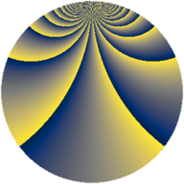# Properties

 Label 1380.2.sLevel $1380$ Weight $2$ Character orbit 1380.s Rep. character $\chi_{1380}(967,\cdot)$ Character field $\Q(\zeta_{4})$ Dimension $264$ Sturm bound $576$

# Related objects

## Defining parameters

 Level: $$N$$ $$=$$ $$1380 = 2^{2} \cdot 3 \cdot 5 \cdot 23$$ Weight: $$k$$ $$=$$ $$2$$ Character orbit: $$[\chi]$$ $$=$$ 1380.s (of order $$4$$ and degree $$2$$) Character conductor: $$\operatorname{cond}(\chi)$$ $$=$$ $$20$$ Character field: $$\Q(i)$$ Sturm bound: $$576$$

## Dimensions

The following table gives the dimensions of various subspaces of $$M_{2}(1380, [\chi])$$.

Total New Old
Modular forms 592 264 328
Cusp forms 560 264 296
Eisenstein series 32 0 32

## Trace form

 $$264q + 24q^{8} + O(q^{10})$$ $$264q + 24q^{8} + 16q^{10} + 16q^{12} + 8q^{13} - 32q^{16} + 40q^{17} + 16q^{22} + 40q^{25} - 32q^{26} - 24q^{28} - 16q^{30} - 16q^{33} - 8q^{37} - 32q^{38} + 16q^{40} - 8q^{45} - 16q^{52} - 8q^{53} + 32q^{56} + 8q^{58} - 40q^{65} + 48q^{66} + 32q^{68} + 24q^{70} + 24q^{72} - 88q^{73} + 48q^{76} + 48q^{78} - 264q^{81} + 8q^{82} + 8q^{85} + 32q^{86} - 120q^{88} - 24q^{90} + 40q^{97} + 8q^{98} + O(q^{100})$$

## Decomposition of $$S_{2}^{\mathrm{new}}(1380, [\chi])$$ into newform subspaces

The newforms in this space have not yet been added to the LMFDB.

## Decomposition of $$S_{2}^{\mathrm{old}}(1380, [\chi])$$ into lower level spaces

$$S_{2}^{\mathrm{old}}(1380, [\chi]) \cong$$ $$S_{2}^{\mathrm{new}}(20, [\chi])$$$$^{\oplus 4}$$$$\oplus$$$$S_{2}^{\mathrm{new}}(60, [\chi])$$$$^{\oplus 2}$$$$\oplus$$$$S_{2}^{\mathrm{new}}(460, [\chi])$$$$^{\oplus 2}$$